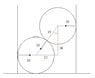# Force reactions of balls in a tube

• Karol

## Homework Statement

Two smooth balls of radius 10[cm] and weight 6[kg] are inside a tube of radius 16[cm] that's on the table. what are the reactions from the walls and what is the minimum mass of the tube to be in equilibrium

## Homework Equations

Disassembling of forces using trigonometry

## The Attempt at a Solution

The middle triangle is a classic 3-4-5 straight angle triangle multiplied by 15 so the reactions from the walls are 3x15=45. but the reactions are horizontal so at any weight the cylinder stays in place, no?

#### Attachments

•Snap1.jpg
9.2 KB · Views: 385
Aren't the balls exerting a moment on the tube?

Chet

Last edited: